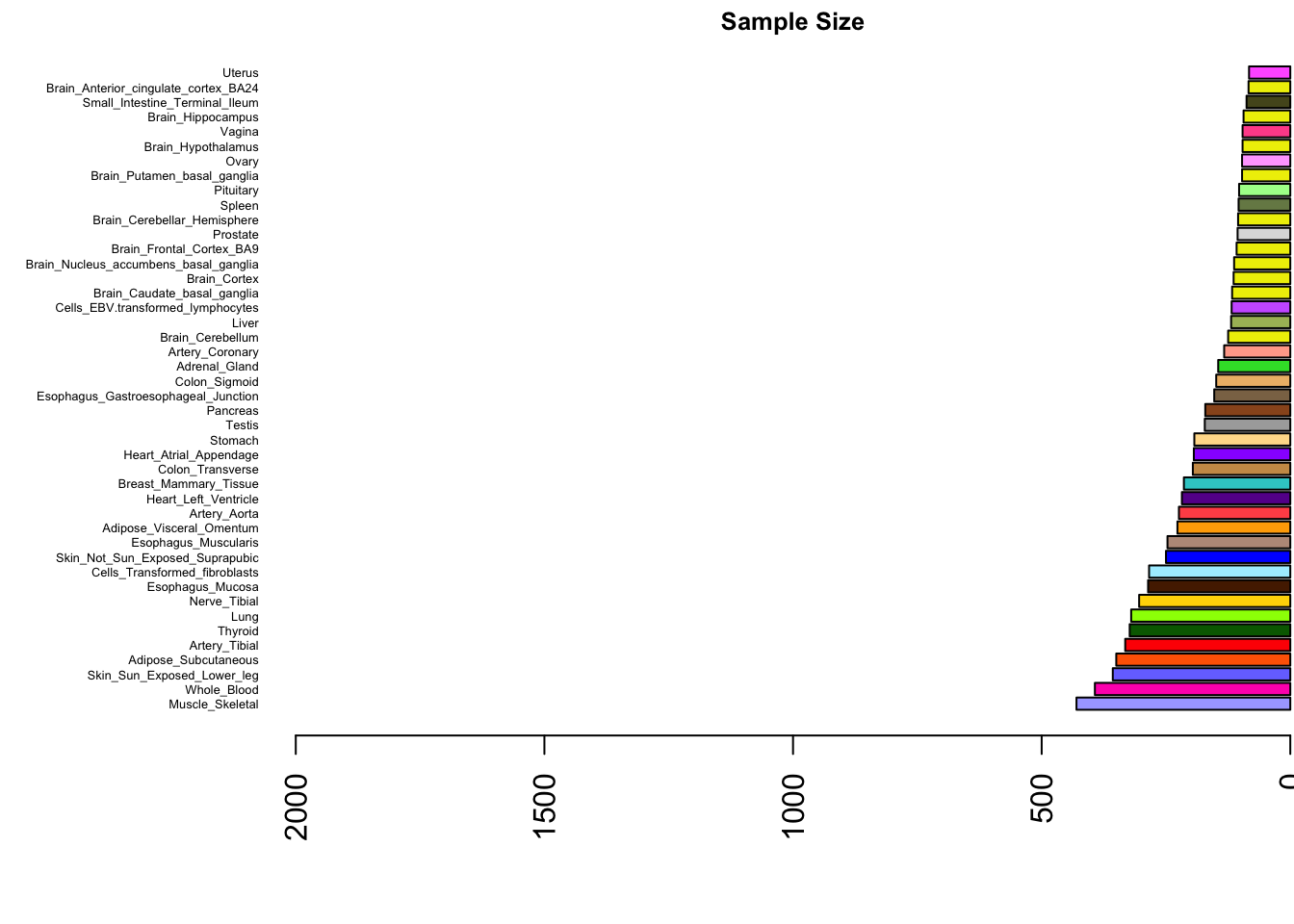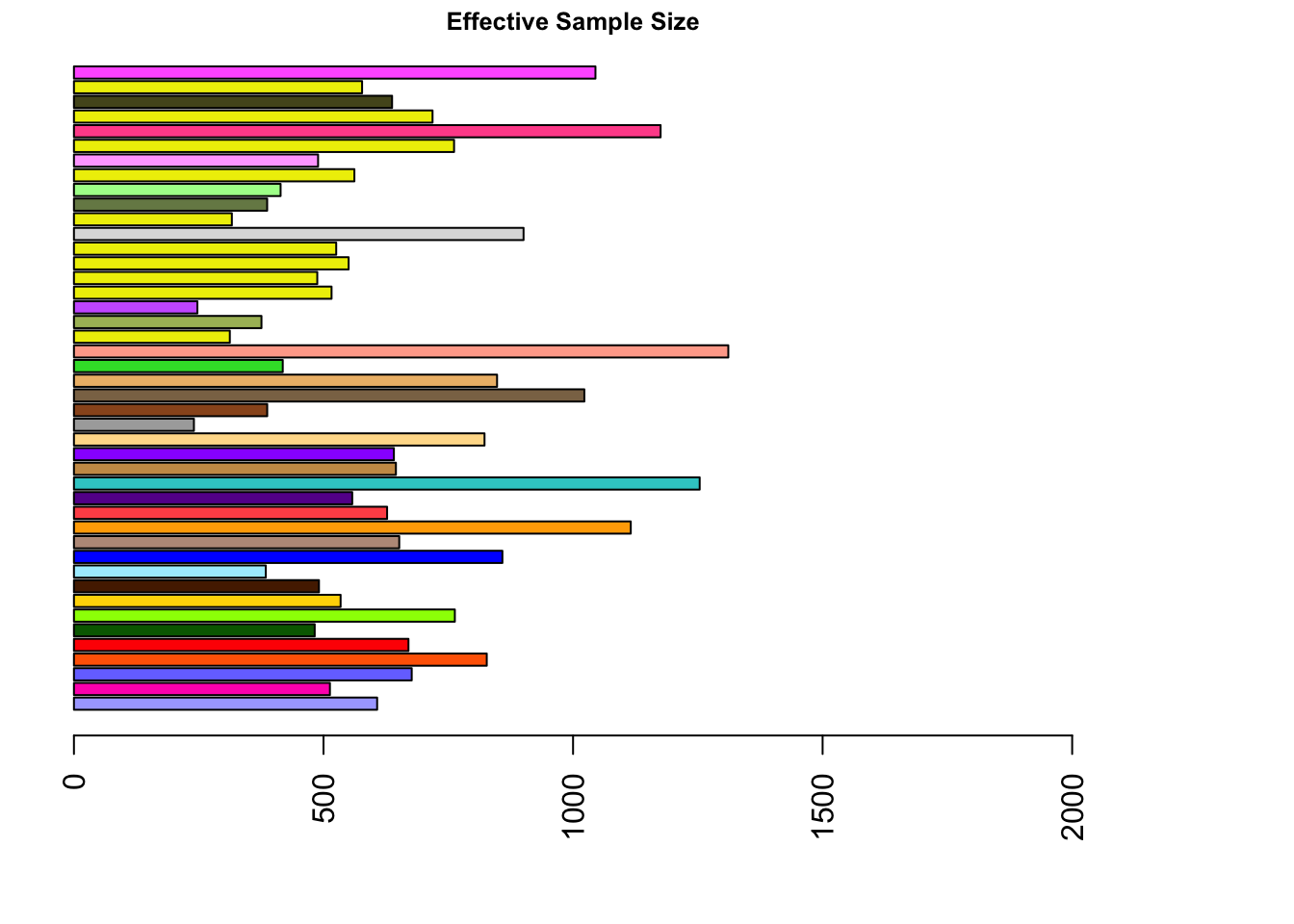The aim of this document is to investigate the correlation of standard error and sample size, and to show how the presence of small sample sizes and large standard errors in biologically ‘unique’ tissues drives incompatibilities between the fold-size sharing heatmap and significance sharing heatmap.

## Warning: package 'knitr' was built under R version 3.2.5

Look at the ordering of Sample Size and see how it is almost identical to that of standard error, though no sample sizes differ by more than about 4 fold.

Now we look at the median posterior variances:

marginal.var=read.table("../../Data_vhat/withvhatmarginal.var.txt")[,-1]

Now let’s plot effective sample size. Recall:

$n_{jeff}=\frac{s_{j}^2}{\tilde{s_{j}^2}}$

WE could also do back to back

original.var=as.matrix(standard.error.from.z)^2
#original.var=(standard.error.from.z/standard.error.from.z)^2
size=as.matrix(exp.sort)
post.var=as.matrix(marginal.var)*standard.error.from.z^2
njeffective=size*original.var/post.var
increase=njeffective/size

gtex.colors=read.table('../../Data/GTExColors.txt', sep = '\t', comment.char = '')[-missing.tissues,2]
missing.tissues=c(7,8,19,20,24,25,31,34,37)

samplesize=apply(size,2,function(x){unique(x)})
sampleorder=order(samplesize,decreasing = T)
median.nj.effective=apply(njeffective,2,median)
median.nj.increase=apply(increase,2,median)

par(mar=c(5.1,8,1.1,0.1))
barplot(samplesize[sampleorder],cex.names=0.4,las=2,col=as.character(gtex.colors[sampleorder]),horiz = T,xlim=c(2000,0))
title("Sample Size",cex.main=0.8)par(mar=c(5.1,2,1.1,6))
barplot(median.nj.effective[sampleorder],cex.names=0.4,las=2,col=as.character(gtex.colors[sampleorder]),horiz = T,names="",xlim=c(0,2000))

title("Effective Sample Size",cex.main=0.8)